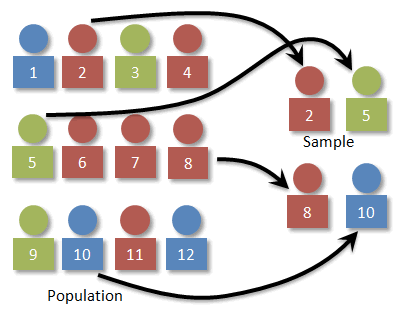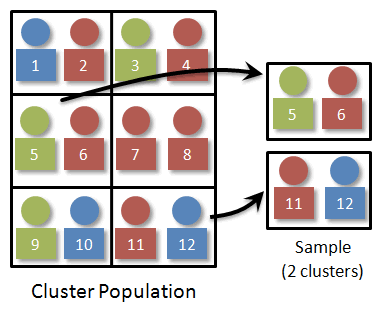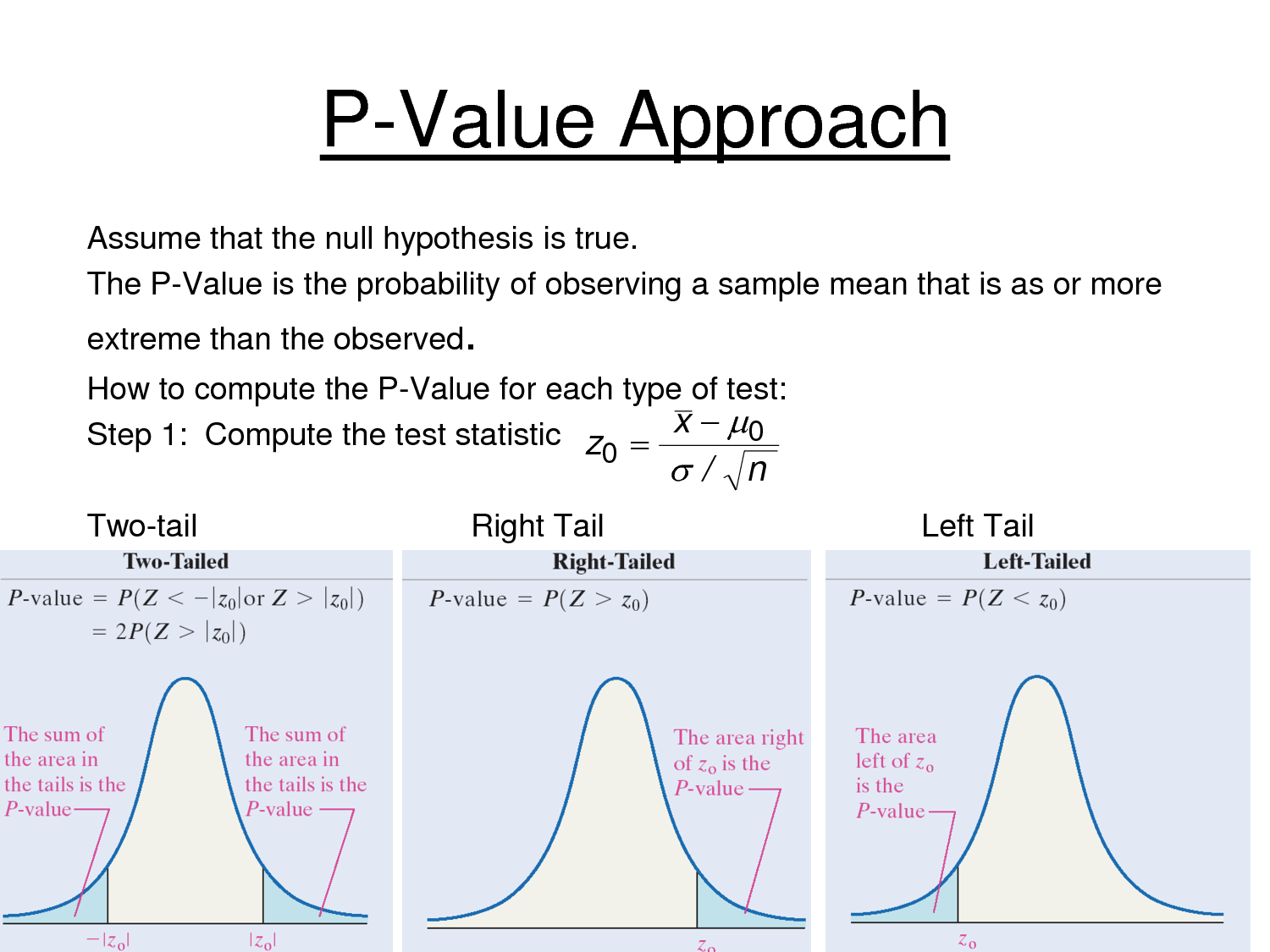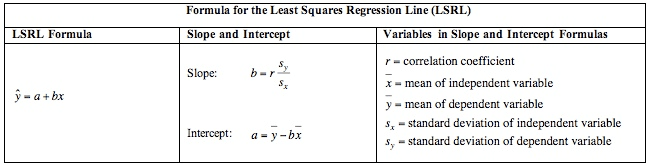# Question 2

• Simple Random Sampling• Stratified Sampling• Systematic Sampling• Cluster Sampling# Question 6# Question 14

• -1 < r < 1# Question 15

• The power of a test is affected by sample size (bigger sample, more power) and alpha level (larger alpha, i.e. .05 compared to .01, more power)

![SIGNIF CANCE LEVEL There is a trade-off between the significance level and power: the more stringent (lower) the significance level, the lower the power. Figure 3 shows that power is lower for the 0.01 level than it is for the 0.05 level. Naturally, the stronger the evidence needed to reject the null hypothesis, the lower the chance that the null hypothesis will be rejected. 1.00 0.80 0.60 0 40 0.20 0.00 a = 0.05 a = 0.01 Figure 3. The relationship between significance level and power with one-tailed tests: u = 75, real u = 80, and o =

1. ](./media/image313.png)

# Question 18